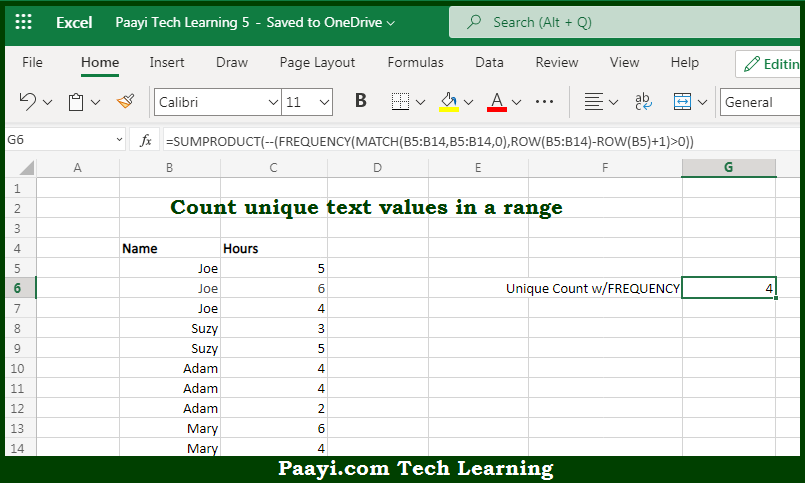# Learn How to COUNT Unique Text Values in a Range in Microsoft Excel

Written by | 0 Comments | 511 Views

In this article, you will learn how to COUNT various things in Microsoft Excel using a single or combination of functions and its purpose. You will also get to know how to COUNT Unique Text Values in a Range and see the generic formula.

COUNT Unique Text Values in a Range in Microsoft Excel

The main purpose of this formula is to count unique text values in a range. Here we will learn how to count unique text values in the given range in Microsoft Excel. That implies, with the help of a formula based on the FREQUENCY, MATCH, ROW, and SUMPRODUCT function you can able to count unique text values in a range. So, with the help of this formula, you can able to count unique text values in the given range.

General Formula to COUNT Unique Text Values in a Range

=SUMPRODUCT(--(FREQUENCY(MATCH(data,data,0),ROW(data)-ROW(data.firstcell)+1)>0))

The Explanation for the COUNT Unique Text Values in a RangeSo we know that with the help of the given formula above you can able to count unique text values in a range. Here we will learn how to count unique text values in the given range in Microsoft Excel. As we know that usually, the formula provided here is more complicated than a similar formula that uses FREQUENCY to count unique numeric values because FREQUENCY doesn't work with non-numeric values. As a result, a large part of the formula simply transforms the non-numeric data into numeric data that FREQUENCY can handle. Working from the inside-out, the MATCH function is used to get the position of each item that appears in the data. So, with the help of this formula, you can able to count unique text values in a range.